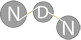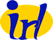NS-3 based Named Data Networking (NDN) simulator ndnSIM 2.5: NDN, CCN, CCNx, content centric networks API Documentation
nfd::RttEstimator Class Reference

implements the Mean-Deviation RTT estimator More...

`#include <rtt-estimator.hpp>`

## Public Types

using Duration = time::microseconds

## Public Member Functions

RttEstimator (uint16_t maxMultiplier=16, Duration minRto=1_ms, double gain=0.1)

void incrementMultiplier ()

void doubleMultiplier ()

Duration computeRto () const

## Static Public Member Functions

static Duration getInitialRtt ()

## Detailed Description

implements the Mean-Deviation RTT estimator

ns3::RttMeanDeviation

This RttEstimator algorithm is designed for TCP, which is a continuous stream. NDN Interest-Data traffic is not always a continuous stream, so NDN may need a different RttEstimator. The design of a more suitable RttEstimator is a research question.

Definition at line 43 of file rtt-estimator.hpp.

## ◆ Duration

 using nfd::RttEstimator::Duration = time::microseconds

Definition at line 46 of file rtt-estimator.hpp.

## ◆ RttEstimator()

 nfd::RttEstimator::RttEstimator ( uint16_t maxMultiplier = `16`, Duration minRto = `1_ms`, double gain = `0.1` )

Definition at line 30 of file rtt-estimator.cpp.

## ◆ getInitialRtt()

 static Duration nfd::RttEstimator::getInitialRtt ( )
inlinestatic

Definition at line 53 of file rtt-estimator.hpp.

 void nfd::RttEstimator::addMeasurement ( Duration measure )

Definition at line 42 of file rtt-estimator.cpp.

References ndn::time::abs().

## ◆ incrementMultiplier()

 void nfd::RttEstimator::incrementMultiplier ( )

Definition at line 61 of file rtt-estimator.cpp.

## ◆ doubleMultiplier()

 void nfd::RttEstimator::doubleMultiplier ( )

Definition at line 67 of file rtt-estimator.cpp.

## ◆ computeRto()

 RttEstimator::Duration nfd::RttEstimator::computeRto ( ) const

Definition at line 73 of file rtt-estimator.cpp.

The documentation for this class was generated from the following files: